Potts model

The Potts model, proposed by Renfrey B. Potts in 1952 , is a generalisation of the Ising model to more than two components. For a general discussion on Potts models see Refs . In practice one has a lattice system. The sites of the lattice can be occupied by particles of different species,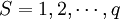$S=1,2, \cdots, q$.

The energy of the system,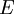$E$, is defined as: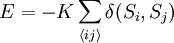$E = - K \sum_{ \langle ij \rangle } \delta (S_i,S_j)$

where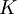$K$ is the coupling constant,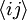$\langle ij \rangle$ indicates that the sum is performed exclusively over pairs of nearest neighbour sites, and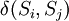$\delta(S_i,S_j)$ is the Kronecker delta. Note that the particular case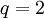$q=2$ is equivalent to the Ising model.

Phase transitions

Considering a symmetric situation (i.e. equal chemical potential for all the species):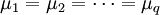$\mu_1 = \mu_2 = \cdots = \mu_q$;

the Potts model exhibits order-disorder phase transitions. For space dimensionality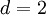$d=2$, and low values of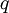$q$ the transitions are continuous (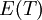$E(T)$ is a continuous function), but the heat capacity,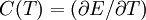$C(T) = (\partial E/\partial T)$, diverges at the transition temperature. The critical behaviour of different values of$q$ belong to (or define) different universality classes of criticality For space dimensionality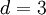$d=3$, the transitions for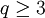$q \ge 3$ are first order ($E$ shows a discontinuity at the transition temperature).